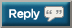# Thread: Let's OHP 2,020,000 lbs in 2020

1. 9 x 40kg (AMRAP)
9 x 40kg (AMRAP)
8 x 40kg (AMRAP)

1040kg (2293lb)

2293 + 823130 = 825,4232. Press (beltless) from yesterdays workout:

58.5kg x 5
61kg x 5
63.5kg x 4
60kg x 5
60kg x 5

Total: 1452kg (3201lb)

3201lb + 825,423 = 828,623lb3. DP and APress 4 x 17 x (35 x 2) = 4760

4760 + 828623 = 833,3834. DP and APress 4 x 17 x (35 x 2) = 4760

4760 + 828623 = 833,383
+ 1,850 = 835,2335. Since 3/3:

3/5: 1820

3/7: 2640

3/12: 1750

3/14: 2340

3/18: 1400 (DB Press as the gym is closed)

3/22: 1440

+11390

Grand Total: 8466236. 5 x 45kg
5 x 45kg
5 x 45kg
6 x 45kg (AMRAP)

945kg (2083lb)

2083 + 846,623 = 848,7067. 5 x 45kg
5 x 45kg
5 x 45kg
6 x 45kg (AMRAP)

945kg (2083lb)

2083 + 846,623 = 848,706
+6600 = 855,3068. DP and APress 4 x 20.5 x (30 x 2) = 4920
4920 + 855306 = 860,2269. DP and APress 4 x 20.5 x (30 x 2) = 4920
4920 + 855306 = 860,226
+1764 = 861,99010.11.12.13. 10 x 40kg
9 x 40kg
9 x 40kg
9 x 40kg
All AMRAP sets

1480kg (3263lb)

3263 + 866,994 = 870,25714. Thanks, it's going to be up to you home gym guys to keep this thread running for a while!15. DP and ArnoldP 4 x 18 x (35 x 2) = 5040
5040 + 870257 = 875,29716. 5 x 45kg
5 x 45kg
5 x 45kg
7 x 45kg

990kg (2182lb)

2182 + 875,297 = 877,47917. Sorry for the absence folks - been on a 10 day deload after some nucleus overload training... will be back at it on Wednesday.18. 5 x 45kg
5 x 45kg
5 x 45kg
7 x 45kg

990kg (2182lb)

2182 + 875,297 = 877,479
+1984 = 879,46319.20. + 3520= 885788

31/03/20 workout21. D Press & A Press 4 x 21 x (30 x 2) = 5040

5040 + 885788 = 890,82822. D Press & A Press 4 x 21 x (30 x 2) = 5040

5040 + 885788 = 890,828
+ 1270 = 892,09823. Back at it! 5,500 + 892,098 = 897,598‬24. Back at it! 5,500 + 892,098 = 897,598‬
+1984 = 899,582

How do you bold number? Just <b > number </b > without spaces?25. +1984 = 899,582

How do you bold number? Just <b > number </b > without spaces?
You put in [ b ] [ /b ] without the spaces like this:-

bold or if on your computer or laptop just hit Ctrl+B and then type whatever you want it will add the B's in automatically same with underlying (U) and italics (I).

Also if you press reply to to any message with the BB code you want to learn e.g. a image then it will show you how they did it e.g. [ img] [/img]26. 30lb KB OHP: 1x5x60=300
40lb KP OHP: 1X5x80=400
60lb Kettlebell OHP: 5x5x120lbs=3000

3700 lb total

899,582+3700=903,28227. You put in [ b ] [ /b ] without the spaces like this:-

bold or if on your computer or laptop just hit Ctrl+B and then type whatever you want it will add the B's in automatically same with underlying (U) and italics (I).

Also if you press reply to to any message with the BB code you want to learn e.g. a image then it will show you how they did it e.g. [ img] [/img]
tyvm28. 30lb KB OHP: 1x5x60=300
40lb KP OHP: 1X5x80=400
60lb Kettlebell OHP: 5x5x120lbs=3000

3700 lb total

899,582+3700=903,282
+1984 = 905,26629. 10 x 40kg
10 x 40kg
9 x 40kg
9 x 40kg
All AMRAP sets

1520kg (3351lb)

3351 + 905,266 = 908,61730. DPress ArnoldPress 4 x 18 x (35 x 2) = 5040

5040 + 908617 = 913,657#### Posting Permissions

• You may not post new threads
• You may not post replies
• You may not post attachments
• You may not edit your posts